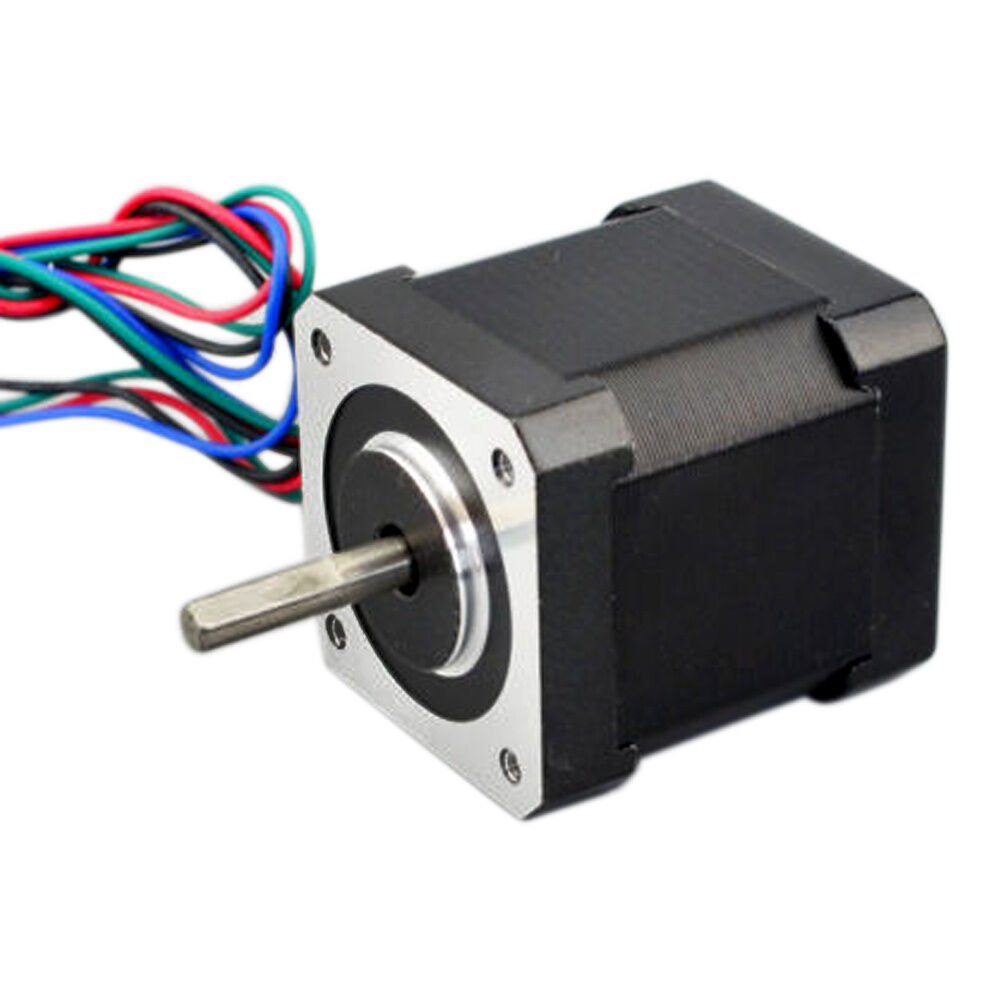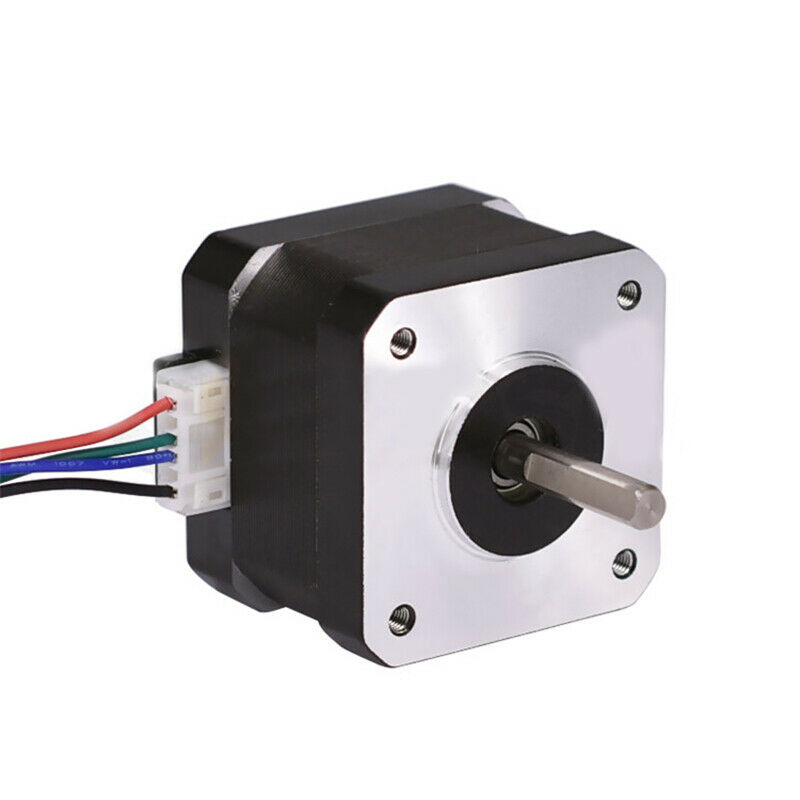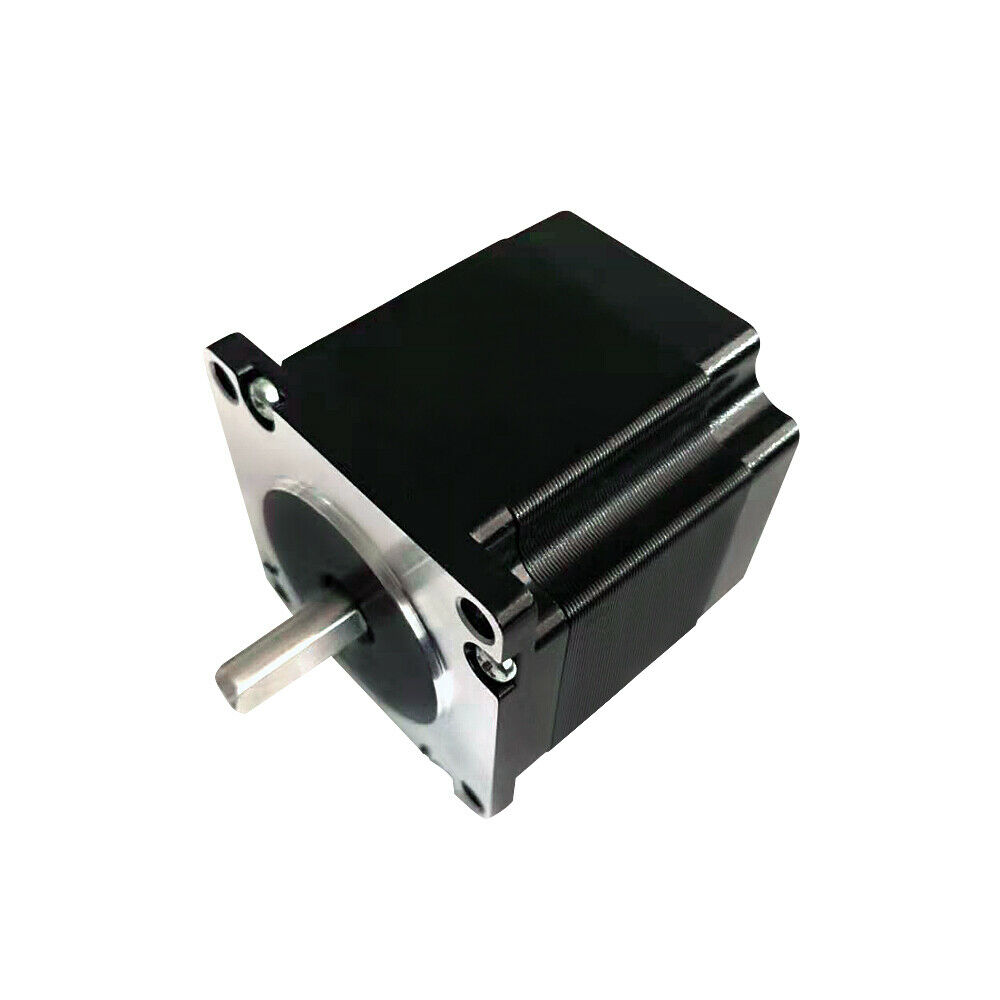# Stepper Motor configurationAll stepper motor are handled by `StepperMotor` class. The class implements:

• Stepper motor FOC algorithm
• Motion control loops
• Monitoring
• User communication interface

## Step 1. Creating the instance of the stepper motor

To create a stepper motor instance you need to specify the number of `pole pairs` of the motor.

``````// StepperMotor(  int pp)
// - pp  - pole pair number
StepperMotor motor = StepperMotor( 50 );
``````

Pole pair number

Most of the stepper motors are 200 step per rotation motors making them 50 pole pair motors. In practice you can know the `pole_paris` number by dividing the number of steps per rotation by `4`.

If you are not sure what your `pole_paris` number is. The library provides you an example code to estimate your `pole_paris` number in the examples `find_pole_pairs_number.ino`.

## Step 2. Linking the sensor

Once when you have the `motor` defined and the sensor initialized you need to link the `motor` and the `sensor` by executing:

``````// link the sensor to the motor
``````

Method `linkSensor` is able to link the motor to any sensor implemented in this library. The `sensor` will be used to determine electrical position of the motor for the FOC algorithm as well as for the motion control loops of velocity and position. See the position sensor docs for more info!

Linking is not necessary when using the openloop motion control.

## Step 3. Linking the driver

Once when you have the `motor` defined and the driver initialized you need to link the `motor` and the `driver` by executing:

``````// link the driver to the motor
``````

The `StepperMotor` class expect to receive a `StepperDriver` class instance, implemented by default with the `StepperDriver4PWM` class. The `driver` deals with all the hardware specific operations related to specific microcontroller architecture and driver hardware. See the stepper driver docs for more info!

## Step 4. Configuration

If you choose not to set some of the configuration parameters they will take values defined in the `defaults.h` file. Check the library source code to dig deeper.

### Step 4.1 Modulation type

You can set them by changing the `motor.foc_modulation` variable:

``````// choose FOC modulation
// FOCModulationType::SinePWM;
motor.foc_modulation = FOCModulationType::SinePWM;
``````

`StepperMotor` class has only Sinusoidal PWM modulation implemented for the moment current version.

For more information about the theory of these approaches please and source code implementation check the FOC implementation docs or visit the digging deeper section.

### Step 4.2 Sensor and motor aligning parameters

The voltage used for the motor and sensor alignment set the variable `motor.voltage_sensor_align`:

``````// aligning voltage [V]
// default 6V
motor.voltage_sensor_align = 3;
``````

### Step 4.3 Motion control parameters

There are 3 different closed loop control strategies implemented in the Arduino SimpleFOClibrary:

Additionally SimpleFOClibrary implements two open loop control strategies as well:

You set it by changing the `motor.controller` variable.

``````// set FOC loop to be used
// MotionControlType::torque      - torque control loop using voltage
// MotionControlType::velocity    - velocity motion control
// MotionControlType::angle       - position/angle motion control
// MotionControlType::velocity_openloop    - velocity open-loop control
// MotionControlType::angle_openloop       - position open-loop control
motor.controller = MotionControlType::angle;
``````

Important!

This parameter doesn't have a default value and it has to be set before real-time execution starts.

Each motion control strategy has its own parameters and you can find more about them on motion control docs.

Here is the list of all the motion control configuration parameters:

``````// set control loop type to be used
motor.controller = MotionControlType::angle;

// controller configuration based on the control type
motor.PID_velocity.P = 0.2;
motor.PID_velocity.I = 20;
motor.PID_velocity.D = 0.001;
// jerk control it is in Volts/s or Amps/s
// for most of applications no change is needed
motor.PID_velocity.output_ramp = 1e6;

// velocity low pass filtering time constant
motor.LPF_velocity.Tf = 0.01;

// angle loop controller
motor.P_angle.P = 20;
motor.P_angle.I = 0; // usually set to 0  - P controller is enough
motor.P_angle.D = 0; // usually set to 0  - P controller is enough
// acceleration limit
motor.P_angle.output_ramp = 1e6;

// motion control limits
// angle loop velocity limit
motor.velocity_limit = 50;
// voltage limit
motor.voltage_limit = 12; // Volts -  default driver.voltage_limit
``````

### Step 4.4 Configuration done - `motor.init()`

Finally the configuration is terminated by running `init()` function which prepares all the hardware and software motor components using the configured values.

``````// initialize motor
motor.init();
``````

## Step 5. Field Oriented Control initialization

After the motor and sensor are initialized and configured, and before we can start the motion control we need to initialize the FOC algorithm.

``````// align sensor and start FOC
motor.initFOC();
``````

Skip for openloop control!

This function should not be called if the motor is run in open loop!

This function aligns sensor and motor zero positions and initializes FOC variables. It is intended to be run in the `setup` function of the Arduino. After the call of this function FOC is ready and our setup is done!

If you are using absolute sensors such as magnetic sensors, in the `initFOC()` you can provide the sensor offset `zero_electric_offset` and sensor direction `sensor_direction` to avoid alignment procedure:

``````// align sensor and start FOC
//motor.initFOC(zero_electric_offset, sensor_direction);
motor.initFOC(2.15, Direction::CW);
``````

You can find these values by running the `find_sensor_offset_and_direction.ino` example.

For more info about what really happens in `initFOC()` check the FOC source code implementation.

## Step 6. Real-time motion control

The real-time motion control of theArduino SimpleFOClibrary is realized with two functions: `motor.loopFOC()` and `motor.move(float target)`.

``````// Function running FOC algorithm in real-time
// it calculates the gets motor angle and sets the appropriate voltages
// to the phase pwm signals
// - the faster you can run it the better Arduino UNO ~1ms, Bluepill ~ 100us
motor.loopFOC();
``````

Skip for openloop control!

This function should not be called if the motor is run in open loop!

The function `loopFOC()` gets the current motor angle from the sensor, turns it into the electrical angle and transforms the quadrature Uq voltage `motor.voltage_q` to the appropriate phase voltages ua, ub and uc which are set then set to the motor.

The transformation of the Uq to ua, ub and uc is defined with the configuration parameter `motor.foc_modulation` that is set to Sinusoidal PWM.

New Feature - Ud direct voltage support!

Sinusoidal PWM supports the direct voltage setting Ud setting. Direct voltage can be set by changing the variable `motor.voltage_d`! This is a new feature and it will be exploited more in the feature releases with the current control.

This function is execution time is critical, therefore it is very important that the `motor.loopFOC()` function is executed as fast as possible!

Rule od thumb: execution time

The faster you can run this function the better!
• Arduino UNO `loop()` ~ 1ms
• Esp32 `loop()` ~ 500us
• Bluepill `loop()` ~ 200us
• Nucleo `loop()` ~ 100us

Finally, when we can set the phase voltages to the motor using the FOC algorithm we can proceed to the motion control. And this is done with `motor.move()` function.

``````// Function executing the control loops configured by the motor.controller parameter of the motor.
// - This function doesn't need to be run upon each loop execution - depends of the use case
//
// target  Either voltage, angle or velocity based on the motor.controller
//         If it is not set the motor will use the target set in its variable motor.target
motor.move(target);
``````

The `move()` method executes the control loops of the algorithm. If is governed by the `motor.controller` variable. It executes either pure voltage loop, velocity loop or angle loop.

It receives one parameter `float target` which is current user defined target value.

• If the user runs velocity loop or velocity open-loop, `move` function will interpret `target` as the target velocity vd.
• If the user runs angle loop or angle open-loop, `move` will interpret `target` parameter as the target angle ad.
• If the user runs the voltage loop, `move` function will interpret the `target` parameter as voltage ud.

The `target` parameter is optional and if it is not set, the target value will be set by the public motor variable `motor.target`. The equivalent code would be:

``````motor.target = 2;
motor.move();
``````

And that is it, you have your complete Field Oriented Controlled BLDC motor with motion control.

## User interaction

SimpleFOClibrary implements two types of real-time user interaction:

## Digging deeper

For more theoretical explanations and source code implementations of the FOC algorithm and the motion control approaches check out the digging deeper section.

## Example code

A simple stepper motor torque control using voltage based on the FOC algorithm.

``````/**
* Torque control example using voltage control loop.
*/
#include <SimpleFOC.h>

// Stepper motor instance
StepperMotor motor = StepperMotor( 50 );
// Stepper driver instance
StepperDriver4PWM driver = StepperDriver4PWM(9, 10, 5, 6, 7, 8);
// sensor instance
MagneticSensorI2C sensor = MagneticSensorI2C(AS5600_I2C);

void setup() {

// initialize encoder sensor hardware
sensor.init();
// link the motor to the sensor

// driver config
// power supply voltage [V]
driver.voltage_power_supply = 12;
driver.init();

// set motion control loop to be used
motor.controller = MotionControlType::torque;

// initialize motor
motor.init();
// align sensor and start FOC
motor.initFOC();

// setting target voltage
motor.target = 2;

_delay(1000);
}

void loop() {

// main FOC algorithm function
motor.loopFOC();

// Motion control function
motor.move();
}
``````